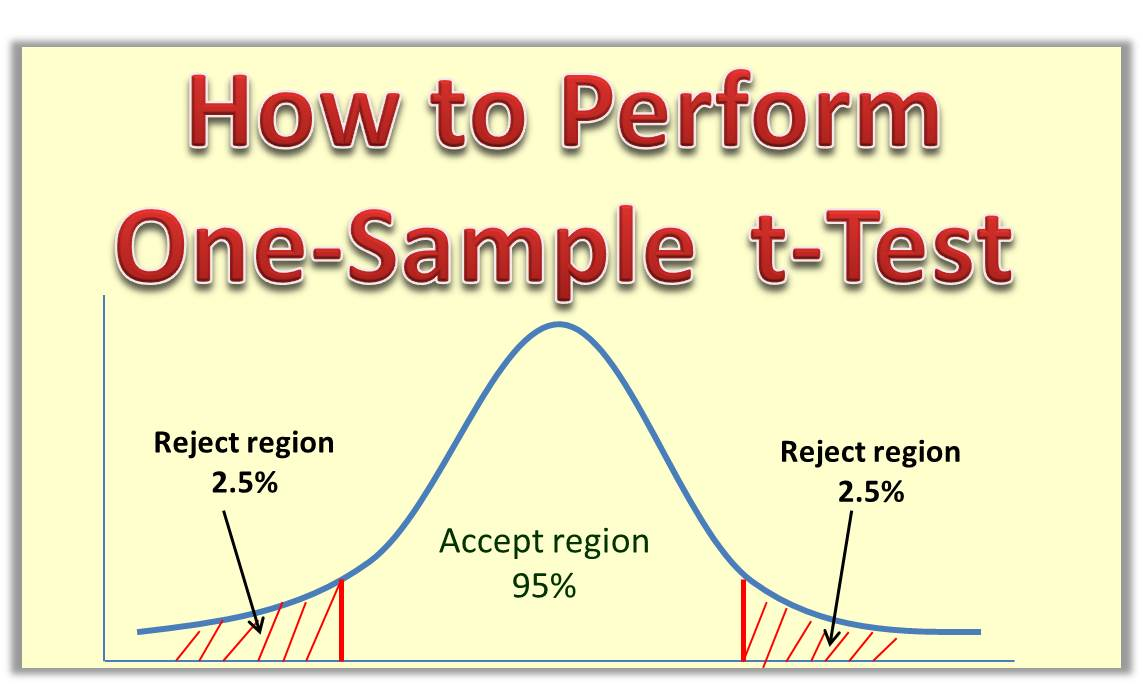# How to Perform One-Sample t-Test Step by StepI am going to teach you how to perform one-sample t-Test, step by step using an example

Question
A research believes that the mean incom of workers in Akokwa town is 20,000 dollars. She wants to test this hypothesis against the alternative that the mean income is not equal to 20,000 naira. A random sample of 9 workers in the town is chosen, and their incomes (in dollars) turn out to be the following:
24000, 13400, 18400, 22900, 13800, 8200, 11100, 9300, 14600.

(a) If the sociologist set a 5 percent significance level, should she accept or reject this hypothesis?
(b) If she sets a 1 percent significance level, shoud she accept or reject the null hypothesis.

Solution
We would start with the first step and progress through the last step. These are the steps:

• Define the null and alternative hypothesis
• State the alpha
• Calculate the degrees of freedom
• State the decision rule (acceptance criteria)
• Calculate the test statistic
• State the results

Step 1: State the null and alternate hypothesis
The null hypothesis is the what is currently believed and needs to be tested. From the question the believe is that the mean income is 20,000 naira. Therefore

H0: mean = 20,000 naira
H1: mean ≠ 20,000 naira

Step 2: State the alpha
From the question, we have 5% significance level. This is the same as alpha level of 0.05 ( or 95% confidence). So we can state the alpha level as:
α = 0.05

Step 3: Calculate the degrees of freedom
Degrees of freedom is given as df = n-1. So in this case:
Degrees of freedom df = 9 -1 = 8

Step 4: State the decision rule (acceptance criteria)
To do this we need to look up the critical value of the t from table of t-distribution or t-Table.
In the table, the alpha is placed in the column while the degrees of freedom are on the rows. In our case we look for df of 8 under 0.05. It is normally written as:
Tcrit or T8, 0.05 = 2.306

Decision rule: Accept the null hypothesis if absolute value of t is less than 2.306 or greater than -2.306

We can do a little sketch to illustrate this in Figure 1.0. Note that the 5% has been divided into two parts of 2.5% on both sides of the graph

Figure 1.0: Sketch of Accept and Reject region

5. Calculate the test Statistic (Absolute value of t)
The formula for the test statistic is given by:

where:
xn is the mean(we need to calculate it)
μ0 is the mean to be tested (given in the question – 20,000)
sd is the standard deviation of the sample (we need to calculate it)
n is the number of elements,  in this case it is 9

xn = Sum/9

Sum =  24000+ 13400+18400+22900+13800+ 8200+11100+9300+14600
Sum =  135700
xn = 135700/9 = 15077.78
sd =  5623.78

Note: Standard deviation is sum of squared deviation divided by n.

Using the formula for t, we can calculate the value of t as: